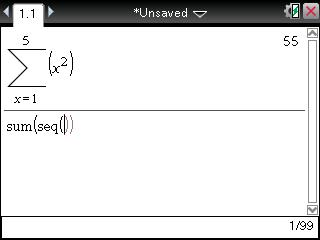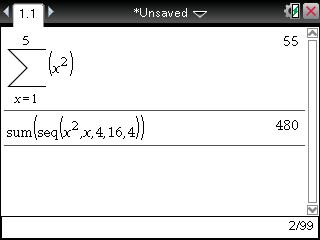# Knowledge Base

## Solution 28870: Calculating the Summation of a Geometric Sequence Using the TI-Nspire™ Family Products.

### How do I calculate the summation of a geometric sequence using the TI-Nspire family products?

To calculate the summation of a geometric sequence using the TI-Nspire family handheld and computer software, the "summation (å)" function must be used. If the summation requires a "step" value, then the "sum" and "seq"  functions must be used together. For help with calculations using either method, follow the examples below. Texas Instruments recommends all TI-Nspire family users update the handheld and computer software to the latest operating system. More information on the latest operating system can be found at the TI-Nspire family latest software website.

Example: Calculate the summation of the geometric sequence x2 as x goes from 1 to 5.

1) Press [home]  to create a New Document. If prompted to save the existing document, choose "Yes" or "No". Press  to insert the Calculator application.
2) Press [menu]   to select the "Sum" function. This will paste the summation (å) function into Calculator application.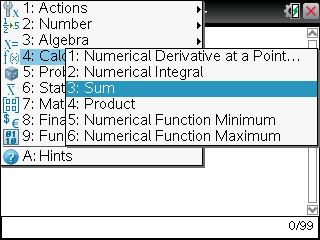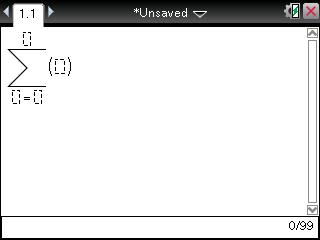3) The cursor will blink in the lower-left box. Input "x", press [tab] to move to the box to the right, then input "1".
4) Press [tab] to move to the top box. Input "5" and press [tab]. The cursor will move to the outside of the summation (å) function.
5) Input x2 and press [enter]. The handheld will display 55 since 12 + 22 + 32 + 42 + 52 = 55.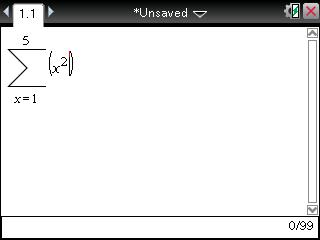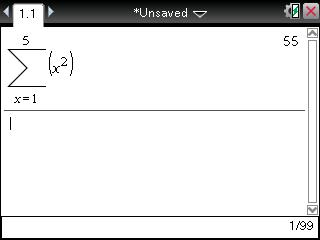Please Note: The next example is also calculated using the Calculator application.

Example: Calculate the summation of the geometric sequence x2 as x goes from 4 to 16 with a step value of 4.

1) Press [menu]    to paste the sum function into the Calculator application. Press [menu]    to paste the seq function into the Calculator application.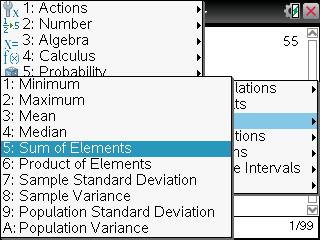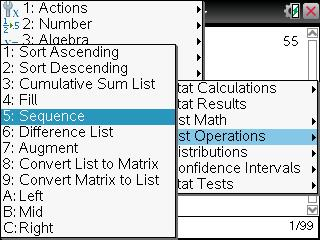2) Inside the second set of parentheses, input "x2,x,4,16,4" and press [enter]. The handheld will return 480 since 42 + 82 + 122 + 162 = 480.